Worksheets and No Prep Teaching Resources
Math Worksheets

# Volume Worksheets

These intriguing worksheets about volume will fill students with a complete understanding of the concepts of more, less, and the units of volume in both the English and metric systems. Students will be thrilled to learn how to calculate the volume and surface areas of all kinds of shapes including prisms, cylinders, cones, pyramids, and spheres. Handy review sheets give students a complete overview of this topic for total mastery of volume and surface area!Volume Capacity: Circle the Bottle with More or with Less Water
Easier: Bottle Sizes the Same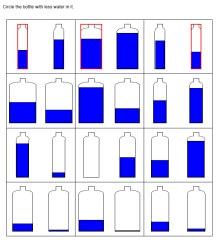Circle the Bottle with More (answers on first row are given) Circle the Bottle with Less (answers on first row are given) Each page will randomly pick either More or Less (answers on first row are given)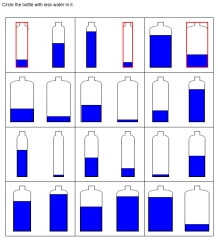Circle the Bottle with More Circle the Bottle with Less Each page will randomly pick either More or Less

More Difficult: Different Size Bottles in Problems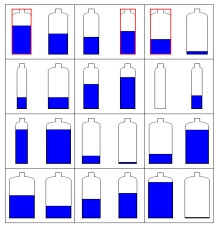Circle the Bottle with More (answers on first row are given) Circle the Bottle with Less (answers on first row are given) Each page will randomly pick either More or Less (answers on first row are given)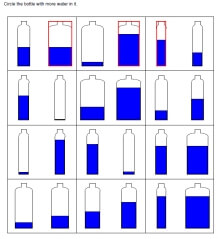Circle the Bottle with More Circle the Bottle with Less Each page will randomly pick either More or Less

Volume of a Cube (Grades 4-6)
Centimeters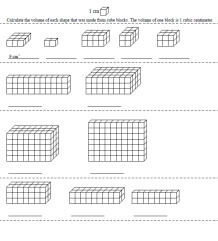Cubic Centimeters: Calculate the volume of the cube (one answer given) Cubic Centimeters: Calculate the volume of the cube

InchesCubic Inches: Calculate the volume of the cube (one answer given) Cubic Inches: Calculate the volume of the cube

Volume and Surface Area
Surface area and volume of right rectangular prisms
Volume of prisms and cylinders (Graphics)
Volume of cones, pyramids, and spheres (Graphics)
Volume of prisms, cylinders, cones, pyramids, and spheres
Surface area of prisms and cylinders
Surface area of pyramids, cones, and spheres
Volume and Surface Area Review Printable

Have a suggestion or would like to leave feedback?